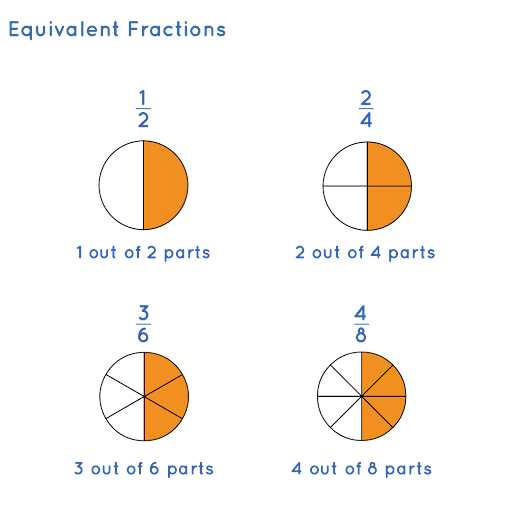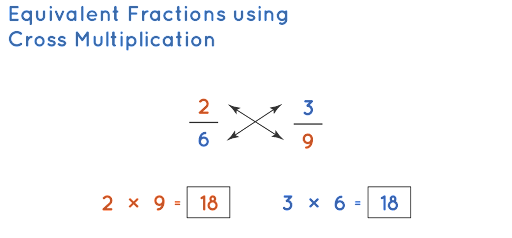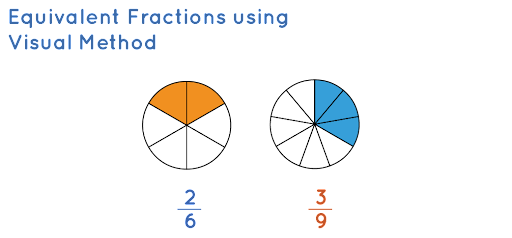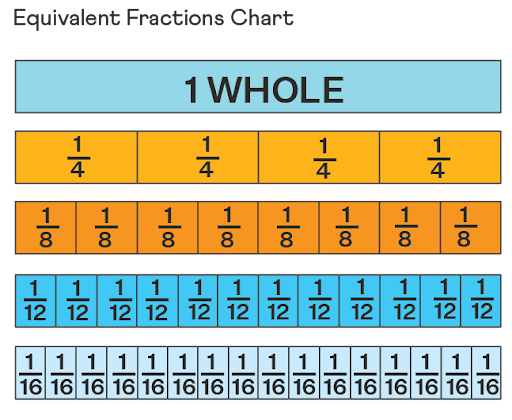Equivalent Fractions | Motivational and Educational Videos for Kids - EasyShiksha

# Equivalent Fractions | Educational Videos

Hello boys and girls how are you voice today we are going to talk about equivalent fractions so kids here we go, Equivalent fractions can be defined as fractions that may have different numerators and denominators but they represent the same value. For example, 9/12 and 6/8 are equivalent fractions because both are equal to 3/4 when simplified.

All equivalent fractions get reduced to the same fraction in their simplest form as seen in the above example. Explore the given lesson to get a better idea of how to find equivalent fractions and how to check if the given fractions are equivalent

### What are Equivalent Fractions?

Two or more fractions are said to be equivalent if they are equal to the same fraction when simplified. For example, the equivalent fractions of 1/5 are 5/25, 6/30, and 4/20, which on simplification, result in the same fraction, that is, 1/5.

##### Equivalent Fractions Definition

Equivalent fractions are defined as those fractions which are equal to the same value irrespective of their numerators and denominators. For example, both 6/12 and 4/8 are equal to 1/2, when simplified, which means they are equivalent in nature.

##### Equivalent Fractions Examples

Here are some examples of equivalent fractions.

Example: 1/2, 2/4, 3/6, and 4/8 are equivalent fractions. Let us see how their values are equal. We will represent each of these fractions as circles with shaded parts. It can be seen that the shaded parts in all the figures represent the same portion if seen as a whole.Here, we can see that the amount of shaded portion is the same in all the circles. Hence, 1/2, 2/4, 3/6, and 4/8 are equivalent fractions.

### How to Find Equivalent Fractions?

Equivalent fractions can be written by multiplying or dividing both the numerator and the denominator by the same number. This is the reason why these fractions get reduced to the same number when they are simplified. Let us understand the two ways in which we can make equivalent fractions:

• Multiply the numerator and the denominator by the same number.
• Divide the numerator and the denominator by the same number.
##### Multiply the Numerator and Denominator by the Same Number

To find the equivalent fractions for any given fraction, multiply the numerator and the denominator by the same number. For example, to find an equivalent fraction of 3/4, multiply the numerator 3 and the denominator 4 by the same number, say, 2. Thus, 6/8 is an equivalent fraction of 3/4. We can find some other equivalent fractions by multiplying the numerator and the denominator of the given fraction by the same number.

• 3 / 4 = 3 × 34 × 3
• 3 × 34 × 3 = 9 / 12
• 3 / 4 = 3 × 44 × 4
• 3 × 44 × 4 = 12 / 16
• 3 / 4 = 3 × 54 × 5
• 3 × 54 × 5 = 15 / 20

Thus, the equivalent fractions of 3/4 are 6/8, 9/12, 12/16, and 15/20.

##### Divide the Numerator and Denominator by the Same Number

To find the equivalent fractions for any given fraction, divide the numerator and the denominator by the same number. For example, to find an equivalent fraction of 72/108, we will first find their common factors. We know that 2 is a common factor of both 72 and 108. Hence, an equivalent fraction of 72/108 can be found by dividing its numerator and denominator by 2. Thus, 36/54 is an equivalent fraction of 72/108. Let us see how the fraction is further simplified:

• 2 is a common factor of 36 and 54. Thus, 36/54 =
• 36 ÷ 254 ÷ 236 ÷ 254 ÷ 2 = 18/27
• Again, 3 is a common factor of 18 and 27. Thus, 18/27 =
• 18 ÷ 327 ÷ 318 ÷ 327 ÷ 3 = 6/9
• Again, 3 is a common factor of 6 and 9. Thus, 6/9 =
• 6 ÷ 39 ÷ 36 ÷ 39 ÷ 3 = 2/3

Therefore, a few equivalent fractions of 72/108 are 36/54, 18/27, 6/9, and 2/3. Here, 2/3 is the simplified form of 72/108 as there is no common factor (other than 1) of 2 and 3.

### How to Determine if Two Fractions are Equivalent?

We need to simplify the given fractions to find whether they are equivalent or not.

Simplification to get equivalent numbers can be done to a point where both the numerator and denominator should still be whole numbers. There are various methods to identify if the given fractions are equivalent. Some of them are:

• Making the denominators the same.
• Finding the decimal form of both the fractions.
• Cross multiplication method.
• Visual method.

Let us identify whether 2/6 and 3/9 are equivalent fractions by each of these methods.

##### Making the Denominators the Same

The denominators of the fractions, 2/6 and 3/9 are 6 and 9. The Least Common Multiple (LCM) of the denominators 6 and 9 is 18. Let us make the denominators of both fractions 18, by multiplying them with suitable numbers.

• 2 / 6 = 2 × 36 × 3
• 2 × 36 × 3 = 6 / 18
• 3 / 9 = 3 × 29 × 2
• 3 × 29 × 2 = 6 / 18

We can observe that both the fractions are equivalent to the same fraction 6/18. Thus, the given fractions are equivalent.

Note: If the fractions are NOT equivalent, we can check the greater or smaller fraction by looking at the numerator of both the resultant fractions. Hence, this method can also be used for comparing fractions.

##### Finding the Decimal form of Both the Fractions

Let us find the decimal form of both the fractions 2/6 and 3/9 to see if they give the same value.

• 2 / 6 = 0.3333333...
• 3 / 9 = 0.3333333...

The decimal values of both the fractions are the same and hence, they are equivalent.

##### Cross Multiplication Method

To identify whether 2/6 and 3/9 are equivalent, we cross multiply them. If both the products are the same, the fractions are equivalent.Since both the products here are 18, the given fractions are equivalent.

##### Visual Method

Let us represent each of the fractions 2/6 and 3/9 pictorially on identical shapes and check if the shaded portions of both are equal.We can see that the shaded portions of both the circles depict the same value. In other words, it can be seen that the shaded parts in both the figures represent the same portion if seen as a whole. Hence, the given fractions are equivalent.

### Equivalent Fractions Chart

Charts and tables are often used to represent concepts in a better way since they serve as a handy reference for calculations and are easier to understand. Anchor charts and tables, like the one given below, make it easier for the students to understand equivalent fractions. Let us use the following chart to find the equivalent fractions of 1/4.From this chart, we can observe that the equivalent fractions of 1/4 are: 2/8, 3/12, 4/16,...

##### Tips on Equivalent Fractions
• Two fractions are said to be equivalent if their values (decimal/graphical) are the same.
• We usually multiply the numerator and denominator of a fraction by the same number to get its equivalent fraction.
• The 'Cross multiplication method' is used to determine whether any two fractions are equivalent or not.
• 'Making the denominators the same' is another method used to determine if two or more fractions are equivalent.